ISEE Lower Level Quantitative : Operations with fractions and whole numbers

Example Questions

Example Question #121 : Operations With Fractions And Whole Numbers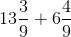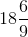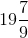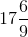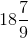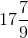Explanation:

When we add mixed numbers, we add whole numbers to whole numbers and fractions to fractions.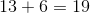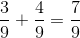Remember, when we are adding fractions we must have common denominators and we only add the numerators.

Example Question #122 : Operations With Fractions And Whole Numbers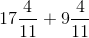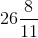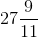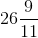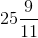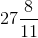Explanation:

When we add mixed numbers, we add whole numbers to whole numbers and fractions to fractions.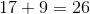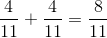Remember, when we are adding fractions we must have common denominators and we only add the numerators.

Example Question #123 : Operations With Fractions And Whole Numbers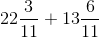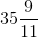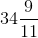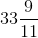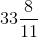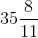Explanation:

When we add mixed numbers, we add whole numbers to whole numbers and fractions to fractions.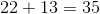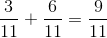Remember, when we are adding fractions we must have common denominators and we only add the numerators.

Example Question #124 : Operations With Fractions And Whole Numbers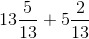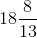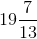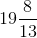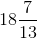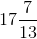Explanation:

When we add mixed numbers, we add whole numbers to whole numbers and fractions to fractions.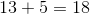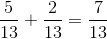Remember, when we are adding fractions we must have common denominators and we only add the numerators.

Example Question #125 : Operations With Fractions And Whole Numbers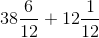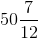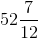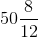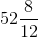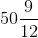Explanation:

When we add mixed numbers, we add whole numbers to whole numbers and fractions to fractions.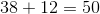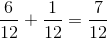Remember, when we are adding fractions we must have common denominators and we only add the numerators.

Example Question #126 : Operations With Fractions And Whole Numbers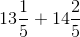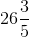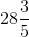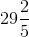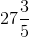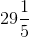Explanation:

When we add mixed numbers, we add whole numbers to whole numbers and fractions to fractions.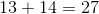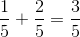Remember, when we are adding fractions we must have common denominators and we only add the numerators.

Example Question #1609 : Ssat Middle Level Quantitative (Math)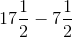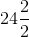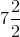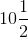Explanation:

When we subtract mixed numbers, we subtract whole numbers by whole numbers and fractions by fractions.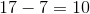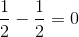Remember, when we are subtracting fractions we must have common denominators and we only subtract the numerators.

Example Question #1610 : Ssat Middle Level Quantitative (Math)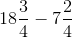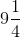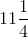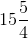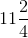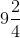Explanation:

When we subtract mixed numbers, we subtract whole numbers by whole numbers and fractions by fractions.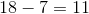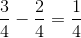Remember, when we are subtracting fractions we must have common denominators and we only subtract the numerators.

Example Question #61 : How To Subtract Fractions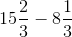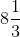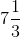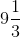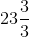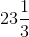Explanation:

When we subtract mixed numbers, we subtract whole numbers by whole numbers and fractions by fractions.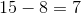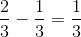Remember, when we are subtracting fractions we must have common denominators and we only subtract the numerators.

Example Question #62 : How To Subtract Fractions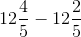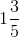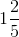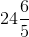Explanation:

When we subtract mixed numbers, we subtract whole numbers by whole numbers and fractions by fractions.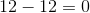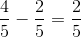Remember, when we are subtracting fractions we must have common denominators and we only subtract the numerators.